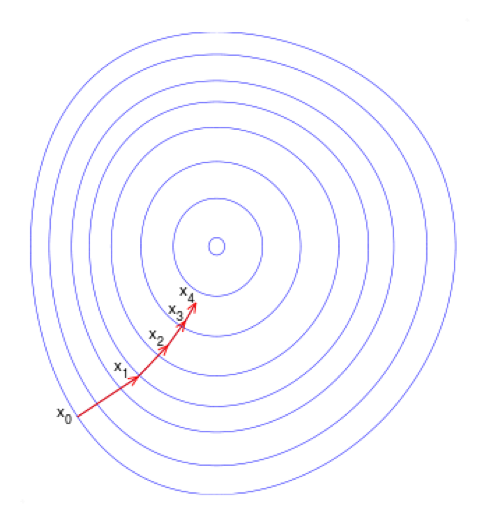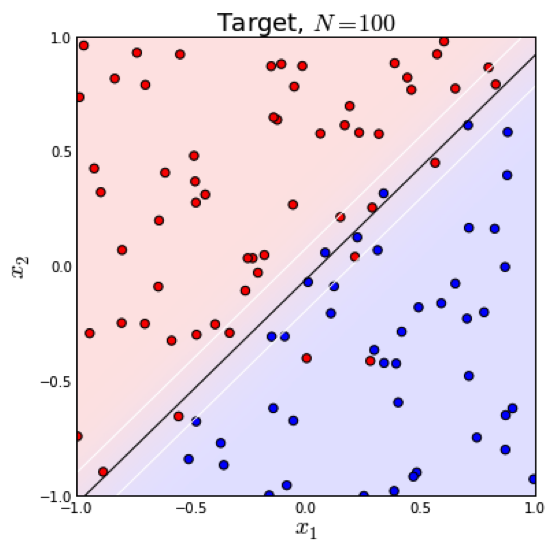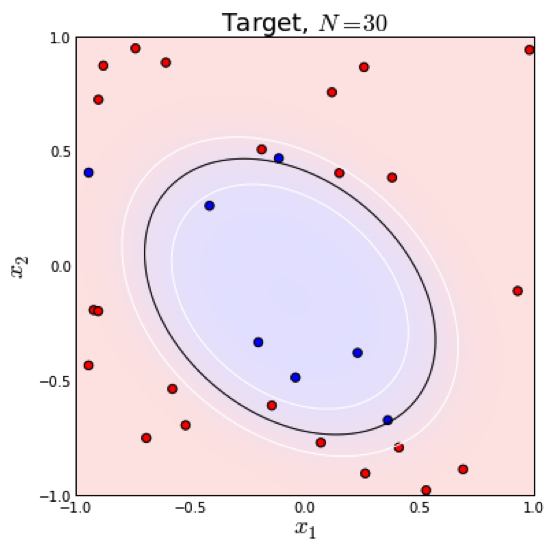# 回归分析之逻辑回归-Logistic Regression

Github：https://github.com/thinkgamer

# Sigmoid函数

## 数学表达式和图形

$f(x)=\frac{1}{1+e^{-x}}$

import math
import matplotlib.pyplot as plt

def sigmoid(x):
return 1 / (1 + math.exp(-x))

# python2 中range生成的是一个数组，py3中生成的是一个迭代器，可以使用list进行转换
X = list(range(-9,10))
Y = list(map(sigmoid,X))

#画图
plt.plot(X,Y)
plt.show()


![sigmoid](https://img-blog.csdn.net/20171219192329328?watermark/2/text/aHR0cDovL2Jsb2cuY3Nkbi5uZXQvR2FtZXJfZ3l0/font/5a6L5L2T/fontsize/400/fill/I0JBQkFCMA==/dissolve/70/gravity/SouthEast)

## LR为什么要使用Sigmoid

$p(y|x) = \frac{P(x|y)P(y)}{\sum{P(x|y)P(y)}}$

$\log\frac{P(y=1|x)}{P(y=0|x)} = \log\frac{P(x|y=1)}{P(x|y=0)} + \log\frac{P(y=1)}{P(y=0)} \ = -\frac{(x-\mu_1)^2}{2\sigma_1^2} + \frac{(x-\mu_0)^2}{2\sigma_0^2}\ + \theta_0$

$\log\frac{P(y=1|x)}{P(y=0|x)} = \theta^T x$

$P(y=1|x) = \frac{e^{\theta^T x}}{1+e^{\theta^T x}} = \frac{1}{1+e^{-\theta^T x}}$

# Logistic Regression 分类器

$P(y=1|x;\theta) = g(\theta^T x) = \frac{1}{1 + e ^ {-\theta^T * x}}$

$y^* = 1, \, \textrm{if} \, P(y=1|x) > 0.5$

# 参数求解

$L(\theta) = P(D|\theta) = \prod P(y|x;\theta) = \prod g(\theta^T x) ^ y (1-g(\theta^T x))^{1-y}$

$l(\theta) = \sum {y\log{g(\theta^T x)} + (1-y)\log{(1-g(\theta^T x))}}$

$-y\log{p(y|x)}-(1-y)\log{1-p(y|x)}$

$J(\theta) = -\frac{1}{N} l(\theta)$

• 选择下降方向（梯度方向，$\nabla {J(\theta)}$
• 选择步长，更新参数 $\theta^i = \theta^{i-1} - \alpha^i \nabla {J(\theta^{i-1})}$
• 重复以上两步直到满足终止条件$\frac{\partial{J}}{\partial{\theta}} = -\frac{1}{n}\sum_i (y_i - y_i^*)x_i + \lambda \theta$

# 分类边界# 资料参考

http://blog.csdn.net/han_xiaoyang/article/details/49123419

https://www.cnblogs.com/sxron/p/5489214.html

https://tech.meituan.com/intro_to_logistic_regression.html